# Pathfinding꞉ GBF Algorithm

The next pathfinding algorithm we'll be looking at is called GREEDY BEST FIRST (GBF).
It's essentially a smarter version of the BFS Algorithm I covered in the previous tutorial. So if you haven't read that then you may get a little lost here.

This is what the gbf looks like: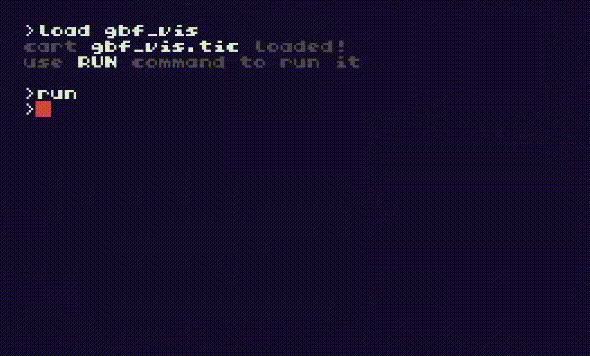The thing that makes GBF smarter is it has a way of deciding what tiles to explore and which to ignore.
And any tiles we can ignore will boost the speed of the pathfinder.

But how does it make that decision?
It uses something known as "Manhattan Distance" to guess which tiles will likely lead to the goal.
Manhattan distance is the number of steps between two points on a graph.
It's calculated like this:

```start={x,y}
goal ={x,y}

distance= math.abs( (start.x-goal.x) + (start.y-goal.y) )```

okay...that seems real smart and all but how does it work?!
Well: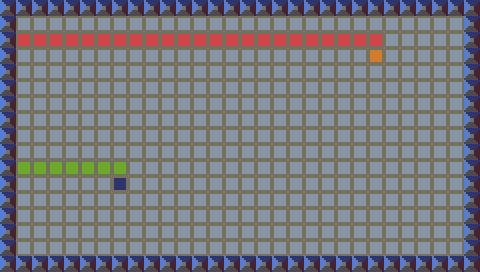Here we have two points, Orange and Blue. Their coordinates are:

```orange={23,3 }
blue={ 7,11}```

Their X distances are shown in red and green respectively.
When we do:

`orange.x-blue.x`

We end up with the horizontal distance between them (shown in yellow):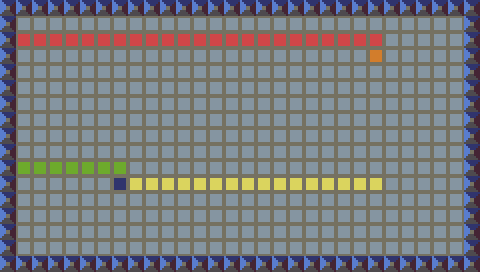that is:

`23-7=16`

and if you count the yellow tiles, you will find that blue would have to take 16 steps to be directly below orange!

And then we do the same with the Y coordinates: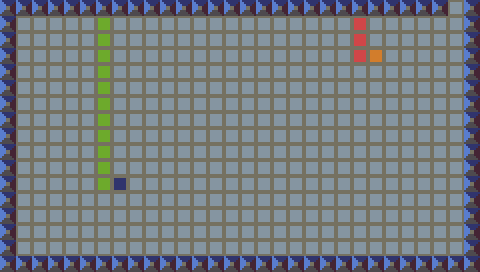Here the Y coordinates are shown in red and green.

And again we subtract one from the other:

`orange.y-blue.y`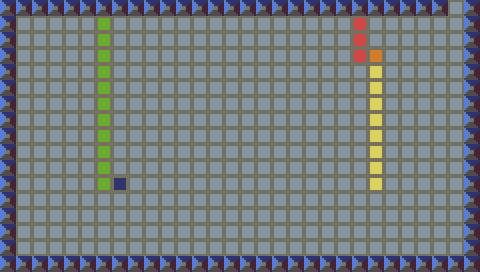to end up with the vertical distance between the two:

`3-11=-8`

but to make this work properly we have to ignore positive and negative numbers so we end up with the absolute value. this is done with the math.abs() function:

`math.abs(3-11)=8`

Now we add them together to end up with the Manhattan Distance:

```math.abs(23-7)=16
math.abs(3-11)=8
8+16=24```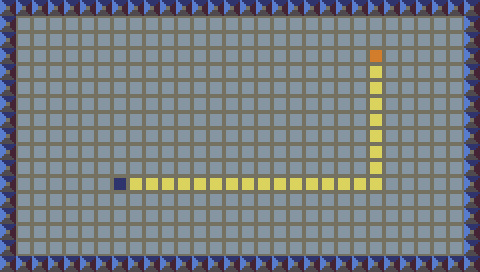And if you start at blue and count every tile you have to move on to get to orange...
You'll find it does in fact take 24 steps to get there.
And it doesn't change anything if you do it in a more direct path: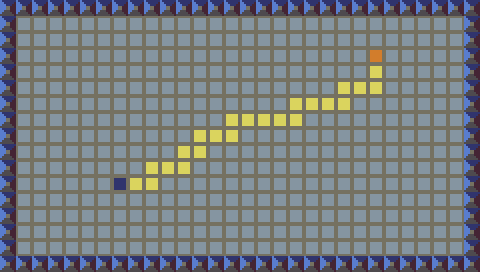This is how Manhattan Distance works.
And This is how we determine a tile's score.

Here's the function I wrote to do this:

``` --a function to estimate a score for a
--tile based on the distance to the goal
function get_score(cell)
local  x=cell
local  y=cell
local gx=goal
local gy=goal
local score=math.abs((x-gx)+(y-gy))
return score
end```

now we can feed any cell we want into this function, and it will return the tile's score.

NOTE
This says it estimates a score.
That's because it has no way of taking obstacles into account.
To do that we'd have to have run another pathfinder first...
And if we've already run a pathfinder then WHY THE HECK ARE WE DOING ALL THIS?!

But a tile simply having a score does nothing useful for us.
Next we need to make the algorithm only explore tiles with the lowest score.
The method I chose to use was to run through the list of open tiles and put the tile with the lowest score at the front.
This is what I call prioritizing the list...or making the best tiles called first.
Here's the function that does that:

``` --a function to place the lowest score
--item at the front of the list
function prioritize(list)
for i,v in ipairs(list) do
local first=list
if v<first then
list=v
list[i]=first
end
end
end```

To understand what's happening, you have to realize that I've built all my tile objects with the score as the fourth item in their list:

`local tile={x_coor, y_coor, parent, score}`

and by calling tile We're retrieving that tile's score.
So we:

1. iterate through all open tiles
2. assign the first tile in the list to first
3. compare every tile's score to the first tile
4. if a tile with a better score is found:
a. swap the two tiles positions.

Now we could have just put the best tile at the front and called it a day...
but that would mean the other tiles would have to shift down:
tile is now tile
tile is now tile
tile is now tile
etc...
This is alot of extra stuff that is really unnecessary!
and every unnecessary calculation we do slows down the program.
So by simply switching them, all the other tiles keep their relative positions.

And with so with the addition of a scoring system (based on manhattan distance)
and a way to prioritize lists based on that score
we can make our pathfinder more efficient:

But remember, this algorithm works by making educated guesses.
So what happens if it guesses wrong?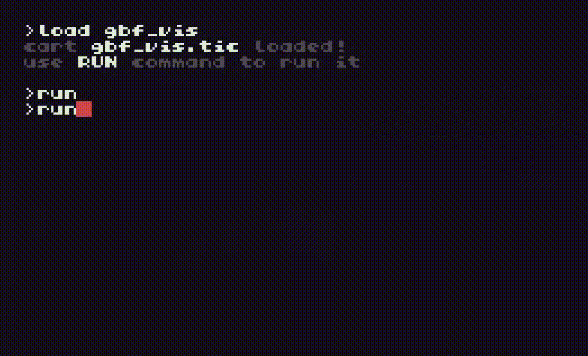The Greedy Best First Algorithm does not guarantee the shortest path!!!
But often enough (especially in simple or open levels) it can return a path twice as fast as the BFS Algorithm.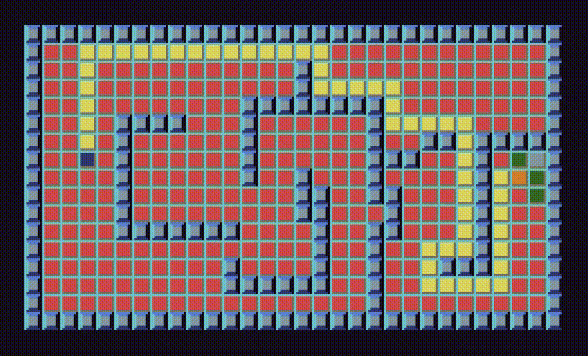This makes it best suited for times when the shortest path isn't needed.
For example:
let's say you have a dungeon crawler.
There's a character that needs to be escorted through the dungeon.
It won't make a difference if he takes the long way, in fact it could give the player more chances to bash some goblins!

Here's the code for the GBF Algorithm:

```-- title:  GBF Pathfinding
-- author: Bear Thorne
-- desc:   Greedy Best First algorithm
-- script: lua

--sprite constants
SPACE=0
FLOOR=1
WALL=17
DUDE=33

OPEN  =2
CLOSED=3

----------------------------------------
------------GBF ALGORITHM---------------
----------------------------------------
function gbf(a,b)

--define start and goal from given points
local start={a.x,a.y,nil,0}
local goal ={b.x,b.y}

--tables to log open/closed cells and
--the shortest path
local open  ={}
local closed={}
local path  ={}

--put the start tile into open and start the search
table.insert(open,#open+1,start)
mode="search"

--a function to check if item "is_in" list
function is_in(item,list)
for i,ele in ipairs(list) do
if ele==item and ele==item then
return true
end
end
end

--a function to place the tile with the
--lowest score at the front of the list
function prioritize(list)
for i,v in ipairs(list) do
local first=list
if v<first then
list=v
list[i]=first
end
end
end

--a function to estimate a score for a
--tile based on the distance to the goal
function get_score(cell)
local  x=cell
local  y=cell
local gx=goal
local gy=goal
local score=math.abs((x-gx)+(y-gy))
return score
end

--a function to get the four adjacents
--to "cell" and log the valid ones
local up   ={cell  ,cell-1}
local down ={cell  ,cell+1}
local left ={cell-1,cell  }
local right={cell+1,cell  }

if not is_in(v,closed) and
not is_in(v,open  ) and
mget(v,v)<WALL then

--build the object for logging
local z={v,v,cell,get_score(v)} --notice the call to get_score() at z
table.insert(open,#open+1,z)
end
end
end

--search code-------------------------
::search::
if #open>0 then
prioritize(open)
current=open
table.remove(open,1)

if current==goal and
current==goal then
local path_end=current

--path building code-----------------
::path_build::
table.insert(path,#path+1,path_end)
if path_end~=start then
path_end=path_end
goto path_build
else
return path
end
end
--------------------------------------

table.insert(closed,#closed+1,current)
if mode=="search" then
goto search
end
end
end
----------------------------------------
---------END OF GBF ALGORITHM-----------
----------------------------------------

--draw onscreen graphics
function draw()
cls()
map(0,0,30,17)
end

function init()
p={x=3 ,y=7}
e={x=27,y=8}
end

init()
function TIC()

path=gbf(p,e)

draw()
for i,v in pairs(path) do
spr(4, v*8,v*8,0)
end

spr(49,p.x*8,p.y*8,0)
spr(50,e.x*8,e.y*8,0)
end```

And the method for implementing it is identical to the way we did the BFS Algorithm in the previous tutorial!

please feel free to use any portion of this code in your own projects. Just throw a credit to me at the top of your file and call it a day! :)

If you have questions, or requests for future tutorials then email me at bearknucklesketching@gmail.com

##### Clone this wiki locally
You can’t perform that action at this time.